February 3, 2014

TradingView Pine Script New Features

It’s been over a month since we launched the first beta version of Pine scripting language. We received a lot of positive responses, constructive criticism, and improvement suggestions. We worked hard and here’s the next update. New features include:

• Inputs for scripted indicators

• Working with the “security” function

• Self Referencing variables

• Multiline functions

• Functions valuewhen and barssince

• Bar coloring of data series – barcolor

• Background coloring – bgcolor

• Fixes the fill function

• Improvements in arithmetic functions

• Automatic type casting

Now let’s look at each feature in more detail.

Inputs for scripted indicators

One of the first features that users asked for was the ability to add inputs to custom scripts, similar to the built-in indicators. You can edit the indicator inputs through the properties window, without needing to edit the Pine script. You can also specify the “title” of the input in the form of a short text string. The title is meant to explain the purpose of the input, and you can specify lowest and highest possible values for numerical inputs.

Instead of writing this in the code:

length = 20
mult = 2.0

You can declare variables length and mult as inputs:

length = input(20, minval=1, title=’Length’)
mult = input(2.0, minval=0.001, maxval=50, title=’Multiplier’)

Further usage of variables length and mult in the script of the indicator is just like any other variable. The indicator properties window now has an Inputs tab, where you can change the input values. The chosen values will be saved as defaults for all new instances of this indicator.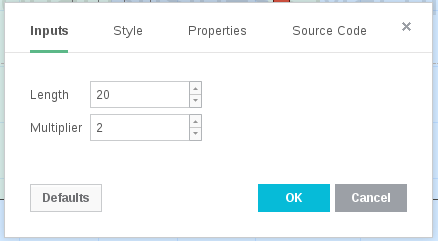We edited the code of all standard scripts and added inputs. For example, here’s the MACD code:

study(title="Moving Average Convergence/Divergence", shorttitle="MACD")
source = close
fastLength = input(12, minval=1), slowLength=input(26,minval=1)
signalLength=input(9,minval=1)
fastMA = ema(source, fastLength)
slowMA = ema(source, slowLength)
macd = fastMA - slowMA
signal = sma(macd, signalLength)
hist = macd - signal
plot(hist, color=red, style=histogram)
plot(macd, color=blue)
plot(signal, color=orange)

First argument of the input function is the default value of the input variable. Other arguments are options. So, for brevity you can write something like this:

i = input(5)
f = input(5.2)
b = input(true)

A more detailed description of all possible function arguments can be found through this link – https://www.tradingview.com/study-script-reference/#fun_input

For now we added inputs of three types: whole numbers, floating point and boolean. Next we plan to add string, expression and symbol/ticker.

Working with the “security” function

Security function (https://www.tradingview.com/study-script-reference/#fun_security) lets you receive data from additional symbols and resolutions, other than the ones to which the indicator is applied. It existed in the first version of Pine Script, but a number of technical difficulties allowed its use in only several of the simplest cases (see standard scripts Compare and Correlation Coeff). We released a number of improvements designed to broaden the possibilities of the security function.

The Advance Decline Line indicator is an example of a more advanced usage of the security function:

sym(s) => security(s, period, close)
difference = (sym("ADVN") - sym("DECN"))/(sym("UNCN") + 1)
adline = cum(difference > 0 ? sqrt(difference) : -sqrt(-difference))

The script requests three securities at the same time. Results of the requests are then added to an arithmetic formula. As a result we have a stock market indicator used by investors to measure the number of individual stocks participating in an upward or downward trend (http://en.wikipedia.org/wiki/Advance%E2%80%93decline_line).

Third parameter of the security function doesn’t have to be a simple open, high, low, close or volume. This can be any arithmetic expression or a function call. For example, with the help of security you can view a minute chart and display an SMA (or any other indicator) based on a any other resolution (i.e. daily, weekly, monthly).

study(title="High Time Frame MA", overlay=true)
src = close, len = input(9)
out = sma(src, len)
out1 = security(tickerid, 'D', out)
plot(out1)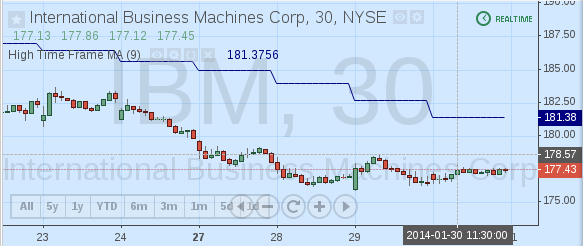Self-Referencing variables

Sometimes it’s necessary to have something in an indicator that stores a status. To solve such issues we created self-referencing variables in Pine Script. A self-referencing variable is a variable that refers to its past values on previous bars from the right side of its declaration. For example:

srv = close + srv + srv

However, on the first bar there is no srv value, so the value srv will be missing on the first bar (to be more precise it will be NaN – Not-A-Number). To change the NaN values it’s possible to turn call to previous values into a nz function (https://www.tradingview.com/study-script-reference/#fun_nz) as follows:

srv = close + nz(srv) + nz(srv)

If you don’t, adding any number with NaN will result in NaN and other calculations won’t work.

With the help of self-referencing variables it’s possible to count all existing bars, although there’s a built-in variable n (https://www.tradingview.com/study-script-reference/#var_n):

study(title="Bar Counter")
barn = na(barn) ? 0 : barn + 1
plot(barn)

Or it’s possible to calculate the moving average without the built-in sma function:

study(title="Custom Simple MA", overlay=true)
len = input(9)
sum = nz(close) + nz(sum) - nz(close[len])
plot(sum/len)

Multiline functions

Pine Script language initially let you declare simple one-line functions, for example:

rnd(val) => val > .99 ? .999 : val < -.99 ? -.999 : val

Of course it’s difficult to do any sort of advanced calculations with just these. So we decided to expand the syntax of declaring functions by making them multiline. Here’s an example of a multiline function:

fun(x, y) =>
a = x * x
b = y * y
sqrt(a + b)

The body of the function is comprised of several statements, each places on a separate line which begins with a tab or 4 spaces (we wanted to make the syntax similar to Python). Statements in the body of the function, except for the last one, must be declarations for variables. The last statement must be an expression, and this will be the value returned by the function. Syntax of one line functions is still supported.

Multiline functions together with self-referencing variables let us build the Connors RSI in Pine Script:

study(title="Connors RSI")
src = close, lenrsi = 3, lenupdown = 2, lenroc = 100
updown(s) =>
isEqual = s == s
isGrowing = s > s
ud = isEqual ? 0 : isGrowing ? (nz(ud) <= 0 ? 1 : nz(ud)+1) : (nz(ud) >= 0 ? -1 : nz(ud)-1)
ud
rsi = rsi(src, lenrsi)
updownrsi = rsi(updown(src), lenupdown)
percentrank = percentrank(roc(src, 1), lenroc)
crsi = avg(rsi, updownrsi, percentrank)
plot(crsi)
band1 = hline(70)
band0 = hline(30)
fill(band1, band0)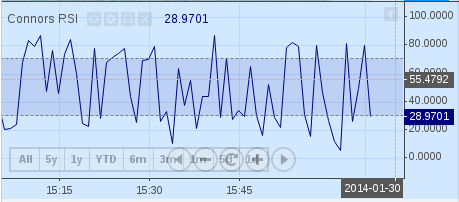We are certain that our users will find lots of uses for multiline functions in Pine Script.

Valuewhen and barssince functions

Barssince function (https://www.tradingview.com/study-script-reference/#fun_barssince) lets you receive the number of bars since some condition is filled, for example when close crossed with SMA.

cross_s = cross(close, sma(close, 20))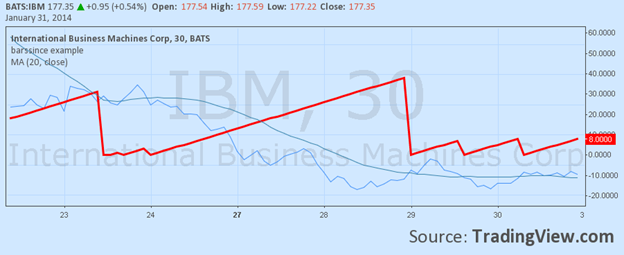Valuewhen function (https://www.tradingview.com/study-script-reference/#fun_valuewhen) returns the value of the specified series at the moment when the condition was filled. For example, the value of the bar when close crossed with SMA.

study(title="valuewhen example", overlay = true)
cross_s = cross(close, sma(close, 20))
plot(valuewhen(cross_s, close, 0), color=red, linewidth=3)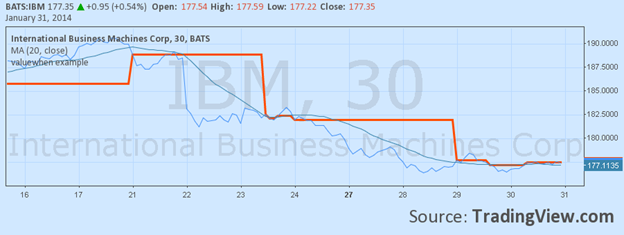Third parameter lets you receive the value after a certain number of times a condition was filled. For example, her the close when it was crossed for the third time (countdown starts at 0, into history)

valuewhen(cross_s, close, 2)

A good example of valuewhen usage is the Divergence Indicator, which you can find in the list of standard scripts.

Barcoloring of series – barcolor

The long-awaited function barcolor lets you specify a color for a bar based on filling of a certain condition. You can use inside and Outside bars in different colors.

study(title="barcolor example", overlay = true)
isUp() => close > open
isDown() => close <= open
isOutsideUp() => high > high and low < low and isUp()
isOutsideDown() => high > high and low < low and isDown()
isInside() => high < high and low > low
barcolor(isInside() ? yellow : isOutsideUp() ? aqua : isOutsideDown() ? purple : na)

As you can seem, when passing the na value, the colors stay the default chart color.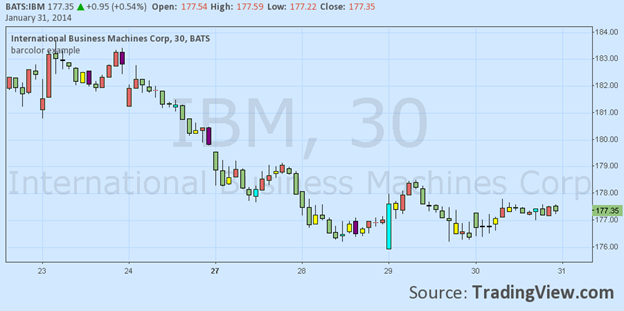Background coloring – bgcolor

Similar to the barcolor function, the background function changes the color of the background. Function will the color of that can be calculated in an expression, and an optional parameter transp – transparency from 0-100, which is 90 by default.

As an example, here’s a script for coloring trading sessions (use it on EURUSD, 30 min resolution)

study(title="bgcolor example", overlay=true)
timeinrange(res, sess) => time(res, sess) != 0
premarket = #0050FF
regular = #0000FF
postmarket = #5000FF
sessioncolor = timeinrange("30", "0400-0930") ? premarket : timeinrange("30", "0930-1600") ? regular : timeinrange("30", "1600-2000") ? postmarket : notrading
bgcolor(sessioncolor, transp=75)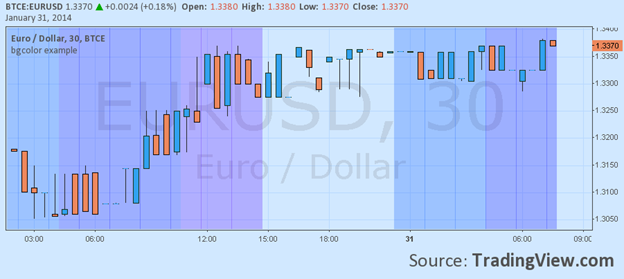Improvement of the fill function

Fill function lets you color the background between two series, or two horizontal lines (created with hline). In its first version, it was significnatly limited – it couldn’t be used more than once per script. That behavior was influenced strictly by technical limitations. Right now there are no limitations for the number of fills, which is shown by the following example:

study(title="Fill Example")
p1 = plot(sin(high))
p2 = plot(cos(low))
p3 = plot(sin(close))
fill(p1, p3, color=green)
fill(p2, p3, color=blue)
h1 = hline(0)
h2 = hline(1.0)
h3 = hline(0.5)
h4 = hline(1.5)
fill(h1, h2, color=yellow)
fill(h3, h4, color=lime)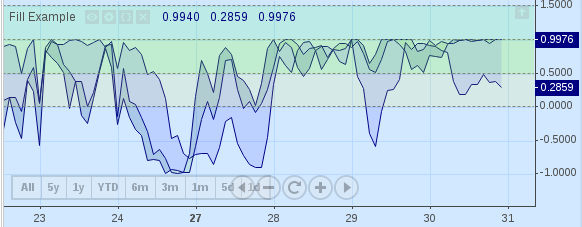Improvements in arithmetic functions

Thanks to our users who write scripts in Pine Script and tell us their feedback here https://getsatisfaction.com/tradingview, we’ve fixed a large number of issues. We added some new functions, which we didn’t have in the past. For example, https://www.tradingview.com/study-script-reference/#fun_round

One of the most common problems is the calculation of the length, and then its usage in functions like sma, wma and others. For example:

length = input(9)
s = sma(close, round(sqrt(length)))
plot(s)

Most arithmetic functions in Pine Script return float (sqrt, exp, log, floor, ceil). However sma requires a second argument for length as a whole numbered value. Now you can get it using the function round.

We noticed the need for a round function in the first version of Pine Script after we tried to add the HullMA indicator.

study(title = "Hull MA", shorttitle="HMA", overlay = true)
source = close, length = input(9, minval=1)
hullma = wma(2*wma(source, length/2)-wma(source, length), round(sqrt(length)))
plot(hullma)

Now you can find the HullMA indicator among other built-in indicators: Open Script Editor, click New and choose HullMA in the list.

Automatic type casting

In the first version of Pine Script it was possible to calculate expressions like this:

s = close + 5
plot(s)

The built-in variable close has a series type. Type of the literal 5 – integer. This resulted in 5 being added to each close price, However, the next code was not performed.

s = 5
plot(s)

But because the plot function demands that the first argument be of the series type. We added the automatic type casting of the integer and float types in to the series type. Now it works.

Conclusion

PineScript – is a young, but fast-growing language meant for traders, We are constantly working on improving existing features, and adding new ones. We will always be happy.to listen to feedback in terms of features and bugs. You can submit bug reports here – https://getsatisfaction.com/tradingview/topics/pine_scripting_language. Thank you for using TradingView!